Open in App
Not now

# Compute element-wise logical AND, OR and NOT of tensors in PyTorch

• Last Updated : 28 Mar, 2022

In this article, we are going to see how to compute element-wise logical AND, OR, and NOT of given tensors in PyTorch. We can compute this by using the torch.logical_and(), torch.logical_or(), and torch.logical_not() methods. Let’s discuss all of them one by one.

## Compute element-wise with logical AND

torch.logical_and() – This method is used to compute the element-wise logical AND of the given tensor. This method treated the non-zero values as True and zero values as False. The following syntax is used to compute logical AND.

Syntax: torch.logical_and(input, other)

Parameters

• input –  This is our input tensor
• other – This tensor is to compute AND with input tensor.

Return : This method returns a tensor with values we get after computing the logical AND.

Example 1:

The following program is to compute element-wise logical AND on two 1D tensors having boolean values.

## Python3

 `# Import the required library ` `import` `torch ` ` `  `# create two tensors having boolean values ` `tens_1 ``=` `torch.tensor([``True``, ``True``, ``False``, ``False``]) ` `tens_2 ``=` `torch.tensor([``True``, ``False``, ``True``, ``False``]) ` ` `  `# display the above created tensors ` `print``(``"Input Tensor 1: "``, tens_1) ` `print``(``"Input Tensor 2: "``, tens_2) ` ` `  `# compute the logical AND of input1 and input2 ` `tens ``=` `torch.logical_and(tens_1, tens_2) ` ` `  `# print result ` `print``(``"\nAfter Compute Logical AND: "``, tens) `

Output: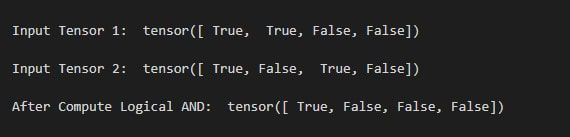Example 2:

The following program is to understand how to compute element-wise logical AND on two 2D tensors.

## Python3

 `# Import the required library ` `import` `torch ` ` `  `# create two tensors ` `tens_1 ``=` `torch.tensor([[``10``, ``0``], [``0``, ``20``]]) ` `tens_2 ``=` `torch.tensor([[``0``, ``30``], [``0``, ``40``]]) ` ` `  `# display the tensors ` `print``(``"Input Tensor 1: \n"``, tens_1) ` `print``(``"Input Tensor 2: \n"``, tens_2) ` ` `  `# compute the logical AND ` `tens ``=` `torch.logical_and(tens_1, tens_2) ` ` `  `# print result ` `print``(``"After Compute Logical AND: \n"``, tens) `

Output: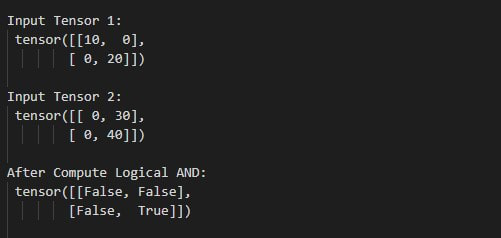## Compute element-wise with logical OR

torch.logical_or() – This method is used to compute the element-wise logical OR of the given tensor. This method also treated the non-zero values as True and zero values as False. The following syntax is used to compute logical OR.

Syntax: torch.logical_or(input, other)

Parameters

• input –  This is our input tensor
• other – This tensor is to compute OR with input tensor.

Return –returns a tensor with values we get after computing the logical OR.

Example 1:

The following program is to know how to compute element-wise logical OR on two 1D tensors.

## Python3

 `# Import the required library ` `import` `torch ` ` `  `# create two tensors ` `tens_1 ``=` `torch.tensor([[``10``, ``0``, ``20``, ``0``]]) ` `tens_2 ``=` `torch.tensor([[``0``, ``30``, ``40``, ``0``]]) ` ` `  `# display the tensors ` `print``(``"\n Input Tensor 1: "``, tens_1) ` `print``(``"\n Input Tensor 2: "``, tens_2) ` ` `  `# compute the logical OR ` `tens ``=` `torch.logical_or(tens_1, tens_2) ` ` `  `# print result ` `print``(``"\n After Compute Logical OR: "``, tens) `

Output: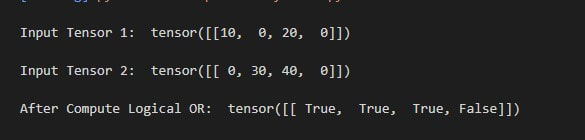Example 2:

The following program is to understand how to compute element-wise logical OR on two 2D tensors.

## Python3

 `# Import the required library ` `import` `torch ` ` `  `# create two tensors ` `tens_1 ``=` `torch.tensor([[``11``, ``0``], [``0``, ``12``]]) ` `tens_2 ``=` `torch.tensor([[``0``, ``13``], [``0``, ``14``]]) ` ` `  `# display the tensors ` `print``(``"\n Input Tensor 1: \n"``, tens_1) ` `print``(``"\n Input Tensor 2: \n"``, tens_2) ` ` `  `# compute the logical OR ` `tens ``=` `torch.logical_or(tens_1, tens_2) ` ` `  `# print result ` `print``(``"\n After Compute Logical OR: \n"``, tens) `

Output: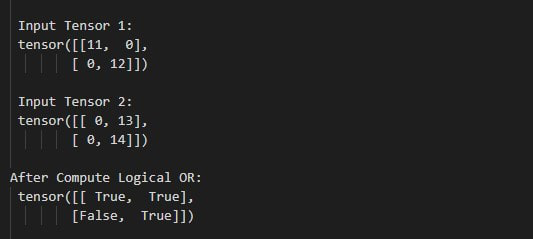## Compute element-wise with logical NOT

torch.logical_not() – This method is used to compute the element-wise logical NOT of the given input tensor. This method also treated the non-zero values as True and zero values as False.  The following syntax is used to compute logical NOT.

Syntax – torch.logical_not(input)

Parameter –

• input – This is our input tensor

Return –This method returns a tensor with values we get after computing the logical NOT.

The following program is to understand how to compute element-wise logical NOT of tensor.

## Python3

 `# import required library ` `import` `torch ` ` `  `# create two tensors ` `tens_1 ``=` `torch.tensor([``11``, ``0``]) ` ` `  ` `  `# display the tensors ` `print``(``"\n Input Tensor 1: \n"``, tens_1) ` ` `  `# compute the logical NOT ` `tens ``=` `torch.logical_not(tens_1) ` ` `  `# display result ` `print``(``"\n After Compute Logical NOT: \n"``, tens) `

Output: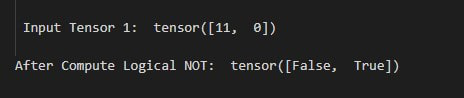My Personal Notes arrow_drop_up
Related Articles